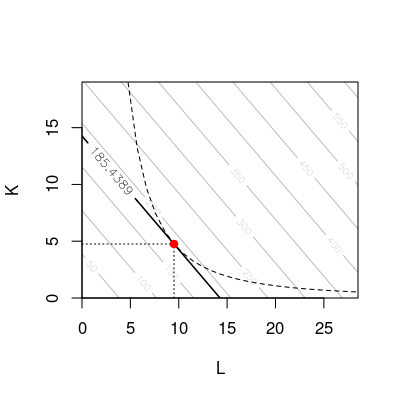## Exam 1

1. #### Question

A firm has the following production function:
 $F\left(K,L\right)=K{L}^{2}.$

The price for one unit of capital is ${p}_{K}=13$ and the price for one unit of labor is ${p}_{L}=13$. Minimize the costs of the firm considering its production function and given a target production output of 430 units.
How high are in this case the minimal costs?

#### Solution

Step 1: Formulating the minimization problem.
 $\begin{array}{ccc}\multicolumn{1}{c}{\underset{K,L}{min}C\left(K,L\right)}& =\hfill & {p}_{K}K+{p}_{L}L\hfill \\ \multicolumn{1}{c}{}& =\hfill & 13K+13L\hfill \\ \multicolumn{1}{c}{\text{subject to:}}& \hfill & F\left(K,L\right)=Q\hfill \\ \multicolumn{1}{c}{}& \hfill & K{L}^{2}=430\hfill \end{array}$

Step 2: Lagrange function.
 $\begin{array}{ccc}\multicolumn{1}{c}{L\left(K,L,\mathit{\lambda }\right)}& =\hfill & C\left(K,L\right)-\mathit{\lambda }\left(F\left(K,L\right)-Q\right)\hfill \\ \multicolumn{1}{c}{}& =\hfill & 13K+13L-\mathit{\lambda }\left(K{L}^{2}-430\right)\hfill \end{array}$

Step 3: First order conditions.
 $\begin{array}{cccc}\multicolumn{1}{c}{\frac{\partial L}{\partial K}}& =\hfill & 13-\mathit{\lambda }{L}^{2}=0\hfill & \hfill \left(1\right)\\ \multicolumn{1}{c}{\frac{\partial L}{\partial L}}& =\hfill & 13-2\mathit{\lambda }K{L}^{2-1}=0\hfill & \hfill \left(2\right)\\ \multicolumn{1}{c}{\frac{\partial L}{\partial \mathit{\lambda }}}& =\hfill & -\left(K{L}^{2}-430\right)=0\hfill & \hfill \left(3\right)\end{array}$

Step 4: Solve the system of equations for $K$, $L$, and $\mathit{\lambda }$.
Equating Equations (1) and (2) after solving for $\mathit{\lambda }$ gives:
 $\begin{array}{ccc}\multicolumn{1}{c}{\frac{13}{{L}^{2}}}& =\hfill & \frac{13}{2K{L}^{2-1}}\hfill \\ \multicolumn{1}{c}{K}& =\hfill & \frac{13}{2·13}·{L}^{2-\left(2-1\right)}\hfill \\ \multicolumn{1}{c}{K}& =\hfill & \frac{13}{26}·L\hfill \end{array}$

Substituting this in the optimization constraint gives:

The minimal costs can be obtained by substituting the optimal factor combination in the objective function:
 $\begin{array}{ccc}\multicolumn{1}{c}{C\left(K,L\right)}& =\hfill & 13K+13L\hfill \\ \multicolumn{1}{c}{}& =\hfill & 61.812955+123.62591\hfill \\ \multicolumn{1}{c}{}& =\hfill & 185.438865\approx 185.44\hfill \end{array}$

Given the target output, the minimal costs are $185.44$.